# ISEE Lower Level Quantitative : Quadrilaterals

## Example Questions

### Example Question #3 : Geometric Measurement: Understanding Concepts Of Area And Relating Area To Multiplication And To Addition

How many square units make up the area of the shape below?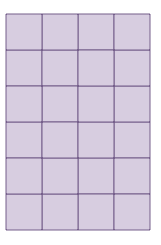Possible Answers: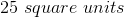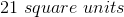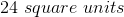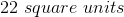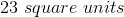Correct answer:Explanation:

The shape is made up of unit squares. We can count the number of squares within the shape to find the area.

There aresquares within the shape.

### Example Question #4 : Geometric Measurement: Understanding Concepts Of Area And Relating Area To Multiplication And To Addition

How many square units make up the area of the shape below?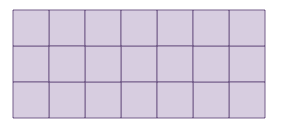Possible Answers: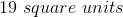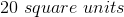Correct answer:Explanation:

The shape is made up of unit squares. We can count the number of squares within the shape to find the area.

There aresquares within the shape.

### Example Question #5 : Geometric Measurement: Understanding Concepts Of Area And Relating Area To Multiplication And To Addition

How many square units make up the area of the shape below?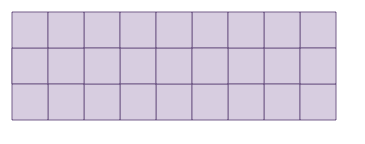Possible Answers: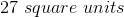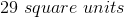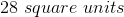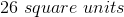Correct answer:Explanation:

The shape is made up of unit squares. We can count the number of squares within the shape to find the area.

There aresquares within the shape.

### Example Question #1 : Geometric Measurement: Understanding Concepts Of Area And Relating Area To Multiplication And To Addition

How many square units make up the area of the shape below?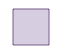Possible Answers: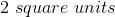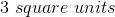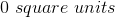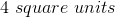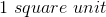Correct answer:Explanation:

The shape is made up of unit squares. We can count the number of squares within the shape to find the area.

There aresquare within the shape.

### Example Question #7 : Geometric Measurement: Understanding Concepts Of Area And Relating Area To Multiplication And To Addition

How many square units make up the area of the shape below?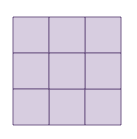Possible Answers: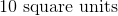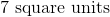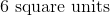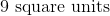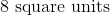Correct answer:Explanation:

The shape is made up of unit squares. We can count the number of squares within the shape to find the area.

There aresquares within the shape.

### Example Question #8 : Geometric Measurement: Understanding Concepts Of Area And Relating Area To Multiplication And To Addition

How many square units make up the area of the shape below?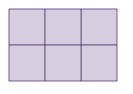Possible Answers: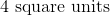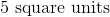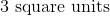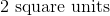Correct answer:Explanation:

The shape is made up of unit squares. We can count the number of squares within the shape to find the area.

There aresquares within the shape.

### Example Question #61 : Quadrilaterals

How many square units make up the area of the shape below?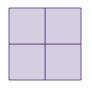Possible Answers:Correct answer:Explanation:

The shape is made up of unit squares. We can count the number of squares within the shape to find the area.

There aresquares within the shape.

### Example Question #291 : Geometry

How many square units make up the area of the shape below?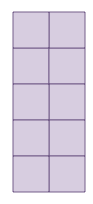Possible Answers: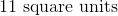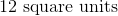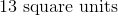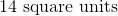Correct answer:Explanation:

The shape is made up of unit squares. We can count the number of squares within the shape to find the area.

There aresquares within the shape.

### Example Question #11 : Geometric Measurement: Understanding Concepts Of Area And Relating Area To Multiplication And To Addition

How many square units make up the area of the shape below?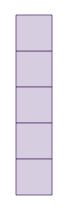Possible Answers: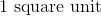Correct answer:Explanation:

The shape is made up of unit squares. We can count the number of squares within the shape to find the area.

There aresquares within the shape.

### Example Question #62 : Quadrilaterals

What is the length of a rectangular room with a perimeter of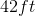and a width of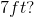Possible Answers: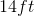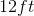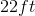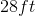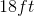Correct answer:Explanation: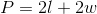We have the perimeter and the width, so we can plug those values into our equation and solve for our unknown.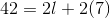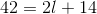Subtractfrom both sides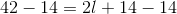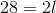Divideby both sides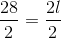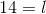### All ISEE Lower Level Quantitative Resources